Courses

# Plane Table Surveying & Calculation Of Area And Volume - 1

## 10 Questions MCQ Test Topicwise Question Bank for GATE Civil Engineering | Plane Table Surveying & Calculation Of Area And Volume - 1

Description
This mock test of Plane Table Surveying & Calculation Of Area And Volume - 1 for Civil Engineering (CE) helps you for every Civil Engineering (CE) entrance exam. This contains 10 Multiple Choice Questions for Civil Engineering (CE) Plane Table Surveying & Calculation Of Area And Volume - 1 (mcq) to study with solutions a complete question bank. The solved questions answers in this Plane Table Surveying & Calculation Of Area And Volume - 1 quiz give you a good mix of easy questions and tough questions. Civil Engineering (CE) students definitely take this Plane Table Surveying & Calculation Of Area And Volume - 1 exercise for a better result in the exam. You can find other Plane Table Surveying & Calculation Of Area And Volume - 1 extra questions, long questions & short questions for Civil Engineering (CE) on EduRev as well by searching above.
QUESTION: 1

Solution:
QUESTION: 2

### The methods used for locating the plane table stations are 1. radiation 2. traversing 3. intersection 4. resection The correct answer is

Solution:

Radiation- All the measurements are taken from a single section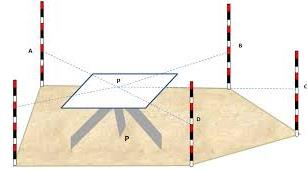Intersection- Plotting same point from two stations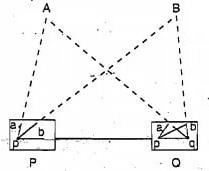Traversing -Traversing by the plane table is similar to compass or theodolite traversing. The method, therefore, can be used for laying down the survey lines of a closed or unclosed traverse.It can be used to locate the plane table stations.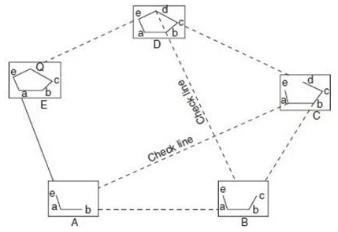Resection: Resection is a method of plane table surveying in which location of plane table is unknown and it is determined by sighting it to known points or plotted points. It is also called method of orientation.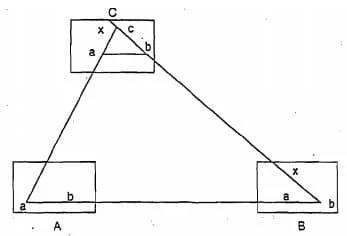QUESTION: 3

### The instrument which is used in plane tabling for obtaining horizontal and vertical distances directly without resorting fo chaining, is known as

Solution:
QUESTION: 4

Orientation of a plane table by solving two point problem is only adopted when

Solution:
QUESTION: 5

For locating an inaccessible point with the help of only a Plane table, one should use

Solution:

(i) Radiation method is suitable only when the area to be surveyed is small and all the stations are visible and accessible from the instrument station.
(ii) Traversing is most suited when a narrow strip of terrain is to be surveyed e.g. survey of roads, railway etc.
(iii) Intersection method is preferred when the distance between stations is large or the stations are inaccessible or the ground is undulating.
(iv) Resection is used when some important details can be plotted easily by choosing any station other than the triangulation station.

QUESTION: 6

Three point problem can be solved by

Solution:
QUESTION: 7

Consider the following statements pertaining to plane table survey
1. Two point problem is solved by mechanical method
2. Three point problem is solved by Bessel’s method
3. In two point problem, auxiliary station is required

Which of these statements is/are correct?

Solution:
QUESTION: 8

Match List-I (Statement) with List-Il (Situation) and select the correct answer using the codes given below the lists:
List-I
A. Accurate centering in plane table surveying is necessary for
B. Exact orientation is more important than accurate centering for
C. The intersection method of plane table surveying is particularly employed for
D. Plane table survey is useful for

List-II
1. Inaccessible points
2. Open country with good intervisibility
3. Large scale maps
4. Small scale maps
5. Hilly regions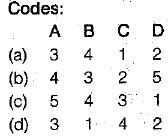Solution:
QUESTION: 9

The method of orienting a plane table with two inaccessible points is known as

Solution:
QUESTION: 10

Match List-l (Methods) with List-II (Procedures) and select the correct answer using the codes given below the lists:
List-I
A. Traversing
B. Resection
C. intersection
D. Radiation

Ltst-ll
1. Rays are drawn to locate the station on which the table is set-up
2. At least two rays are drawn from two different stations to the details to be located
3. Rays are drawn in the direction of details through ine station point on which the table is set-up
4. Rays ace drawn on the map by setting up the table over each of the stations towards the subsequent station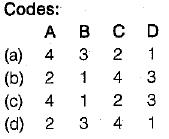Solution: